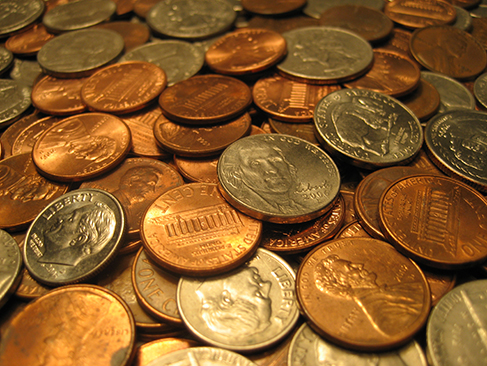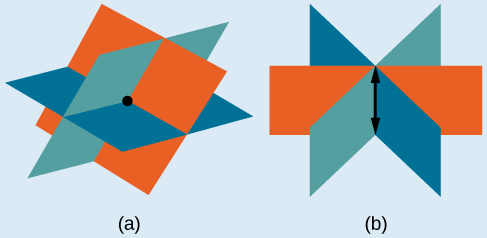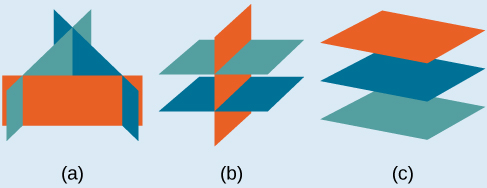# 7.2 Systems of linear equations: three variables

 Page 1 / 8
In this section, you will:
• Solve systems of three equations in three variables.
• Identify inconsistent systems of equations containing three variables.
• Express the solution of a system of dependent equations containing three variables.(credit: “Elembis,” Wikimedia Commons)

John received an inheritance of $12,000 that he divided into three parts and invested in three ways: in a money-market fund paying 3% annual interest; in municipal bonds paying 4% annual interest; and in mutual funds paying 7% annual interest. John invested$4,000 more in municipal funds than in municipal bonds. He earned \$670 in interest the first year. How much did John invest in each type of fund?

Understanding the correct approach to setting up problems such as this one makes finding a solution a matter of following a pattern. We will solve this and similar problems involving three equations and three variables in this section. Doing so uses similar techniques as those used to solve systems of two equations in two variables. However, finding solutions to systems of three equations requires a bit more organization and a touch of visual gymnastics.

## Solving systems of three equations in three variables

In order to solve systems of equations in three variables, known as three-by-three systems, the primary tool we will be using is called Gaussian elimination , named after the prolific German mathematician Karl Friedrich Gauss . While there is no definitive order in which operations are to be performed, there are specific guidelines as to what type of moves can be made. We may number the equations to keep track of the steps we apply. The goal is to eliminate one variable at a time to achieve upper triangular form , the ideal form for a three-by-three system because it allows for straightforward back-substitution to find a solution $\text{\hspace{0.17em}}\left(x,y,z\right),\text{}$ which we call an ordered triple . A system in upper triangular form looks like the following:

The third equation can be solved for $\text{\hspace{0.17em}}z,\text{}$ and then we back-substitute to find $\text{\hspace{0.17em}}y\text{\hspace{0.17em}}$ and $\text{\hspace{0.17em}}x.\text{\hspace{0.17em}}$ To write the system in upper triangular form, we can perform the following operations:

1. Interchange the order of any two equations.
2. Multiply both sides of an equation by a nonzero constant.
3. Add a nonzero multiple of one equation to another equation.

The solution set to a three-by-three system is an ordered triple $\text{\hspace{0.17em}}\left\{\left(x,y,z\right)\right\}.\text{\hspace{0.17em}}$ Graphically, the ordered triple defines the point that is the intersection of three planes in space. You can visualize such an intersection by imagining any corner in a rectangular room. A corner is defined by three planes: two adjoining walls and the floor (or ceiling). Any point where two walls and the floor meet represents the intersection of three planes.

## Number of possible solutions

[link] and [link] illustrate possible solution scenarios for three-by-three systems.

• Systems that have a single solution are those which, after elimination, result in a solution set    consisting of an ordered triple $\text{\hspace{0.17em}}\left\{\left(x,y,z\right)\right\}.\text{\hspace{0.17em}}$ Graphically, the ordered triple defines a point that is the intersection of three planes in space.
• Systems that have an infinite number of solutions are those which, after elimination, result in an expression that is always true, such as $\text{\hspace{0.17em}}0=0.\text{\hspace{0.17em}}$ Graphically, an infinite number of solutions represents a line or coincident plane that serves as the intersection of three planes in space.
• Systems that have no solution are those that, after elimination, result in a statement that is a contradiction, such as $\text{\hspace{0.17em}}3=0.\text{\hspace{0.17em}}$ Graphically, a system with no solution is represented by three planes with no point in common.(a)Three planes intersect at a single point, representing a three-by-three system with a single solution. (b) Three planes intersect in a line, representing a three-by-three system with infinite solutions.All three figures represent three-by-three systems with no solution. (a) The three planes intersect with each other, but not at a common point. (b) Two of the planes are parallel and intersect with the third plane, but not with each other. (c) All three planes are parallel, so there is no point of intersection.

#### Questions & Answers

show that the set of all natural number form semi group under the composition of addition
Nikhil Reply
what is the meaning
Dominic
explain and give four Example hyperbolic function
Lukman Reply
_3_2_1
felecia
⅗ ⅔½
felecia
_½+⅔-¾
felecia
The denominator of a certain fraction is 9 more than the numerator. If 6 is added to both terms of the fraction, the value of the fraction becomes 2/3. Find the original fraction. 2. The sum of the least and greatest of 3 consecutive integers is 60. What are the valu
SABAL Reply
1. x + 6 2 -------------- = _ x + 9 + 6 3 x + 6 3 ----------- x -- (cross multiply) x + 15 2 3(x + 6) = 2(x + 15) 3x + 18 = 2x + 30 (-2x from both) x + 18 = 30 (-18 from both) x = 12 Test: 12 + 6 18 2 -------------- = --- = --- 12 + 9 + 6 27 3
Pawel
2. (x) + (x + 2) = 60 2x + 2 = 60 2x = 58 x = 29 29, 30, & 31
Pawel
ok
Ifeanyi
on number 2 question How did you got 2x +2
Ifeanyi
combine like terms. x + x + 2 is same as 2x + 2
Pawel
x*x=2
felecia
2+2x=
felecia
×/×+9+6/1
Debbie
Q2 x+(x+2)+(x+4)=60 3x+6=60 3x+6-6=60-6 3x=54 3x/3=54/3 x=18 :. The numbers are 18,20 and 22
Naagmenkoma
Mark and Don are planning to sell each of their marble collections at a garage sale. If Don has 1 more than 3 times the number of marbles Mark has, how many does each boy have to sell if the total number of marbles is 113?
mariel Reply
Mark = x,. Don = 3x + 1 x + 3x + 1 = 113 4x = 112, x = 28 Mark = 28, Don = 85, 28 + 85 = 113
Pawel
how do I set up the problem?
Harshika Reply
what is a solution set?
Harshika
find the subring of gaussian integers?
Rofiqul
hello, I am happy to help!
Shirley Reply
please can go further on polynomials quadratic
Abdullahi
hi mam
Mark
I need quadratic equation link to Alpa Beta
Abdullahi Reply
find the value of 2x=32
Felix Reply
divide by 2 on each side of the equal sign to solve for x
corri
X=16
Michael
Want to review on complex number 1.What are complex number 2.How to solve complex number problems.
Beyan
yes i wantt to review
Mark
16
Makan
x=16
Makan
use the y -intercept and slope to sketch the graph of the equation y=6x
Only Reply
how do we prove the quadratic formular
Seidu Reply
please help me prove quadratic formula
Darius
hello, if you have a question about Algebra 2. I may be able to help. I am an Algebra 2 Teacher
Shirley Reply
thank you help me with how to prove the quadratic equation
Seidu
may God blessed u for that. Please I want u to help me in sets.
Opoku
what is math number
Tric Reply
4
Trista
x-2y+3z=-3 2x-y+z=7 -x+3y-z=6
Sidiki Reply
can you teacch how to solve that🙏
Mark
Solve for the first variable in one of the equations, then substitute the result into the other equation. Point For: (6111,4111,−411)(6111,4111,-411) Equation Form: x=6111,y=4111,z=−411x=6111,y=4111,z=-411
Brenna
(61/11,41/11,−4/11)
Brenna
x=61/11 y=41/11 z=−4/11 x=61/11 y=41/11 z=-4/11
Brenna
Need help solving this problem (2/7)^-2
Simone Reply
x+2y-z=7
Sidiki
what is the coefficient of -4×
Mehri Reply
-1
Shedrak

### Read also:

#### Get Jobilize Job Search Mobile App in your pocket Now!

Source:  OpenStax, College algebra. OpenStax CNX. Feb 06, 2015 Download for free at https://legacy.cnx.org/content/col11759/1.3
Google Play and the Google Play logo are trademarks of Google Inc.

Notification Switch

Would you like to follow the 'College algebra' conversation and receive update notifications?ByBy Brooke DelaneyBy OpenStaxBy Brooke DelaneyBy Rohini AjayBy OpenStaxBy Brooke DelaneyBy Yasser IbrahimBy Jemekia WeedenBy Yacoub JayoghliBy OpenStax